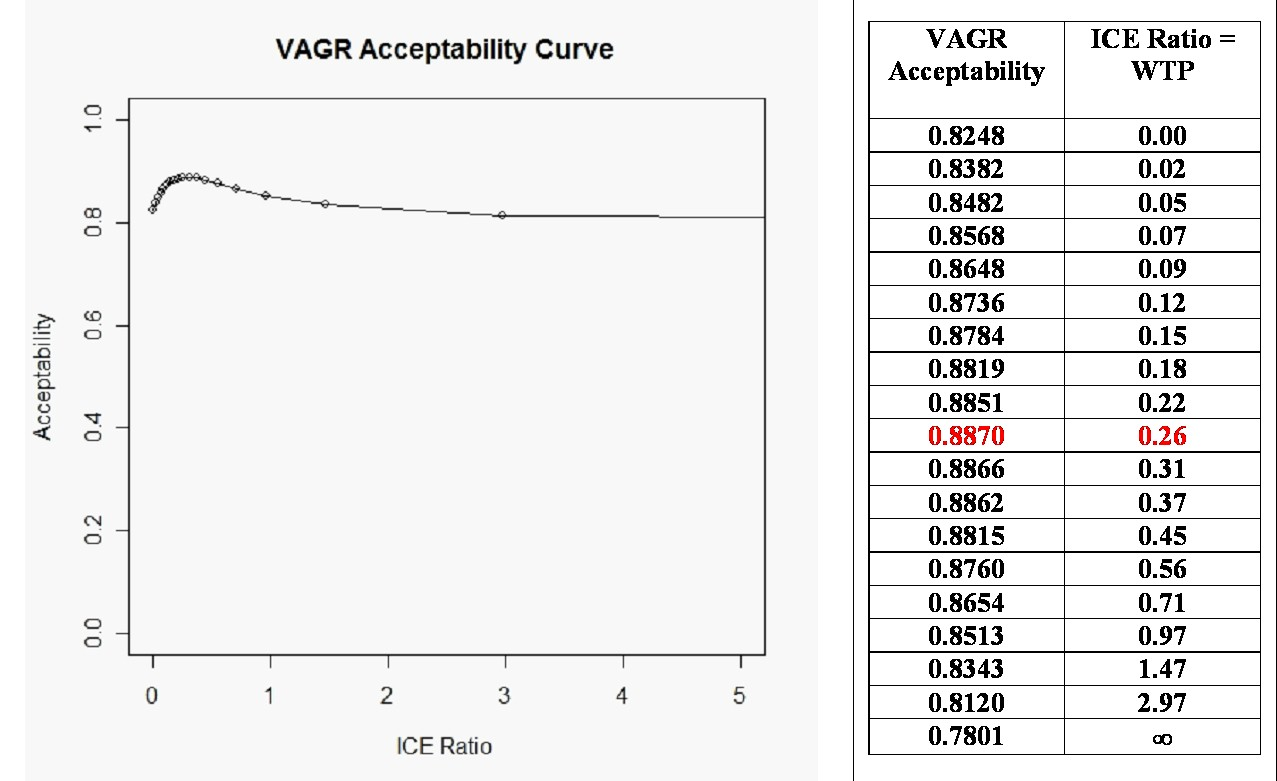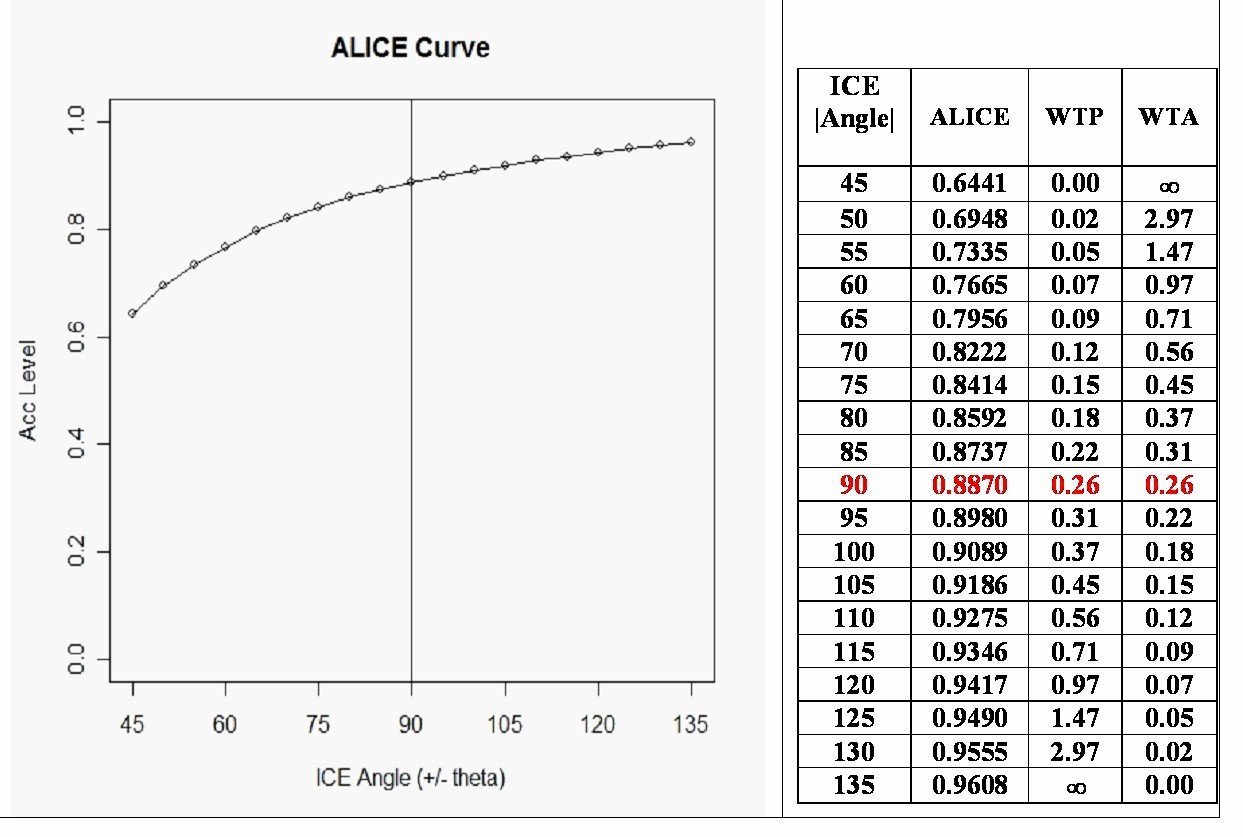### Nav: Back

 ICE Acceptability: VAGR versus ALICE?

The concept of ICE "acceptability" originated in the publication: Van Hout BA, Al MJ, Gordon GS, Rutten FFH.  Costs, effects and C/E ratios alongside a clinical trial.  Health Econ 1994; 3: 309-319.

The alternative (Acceptability Levels In Cost Effectineness) definition is newer; see Figures 7 and 8 of Obenchain RL.  Issues and algorithms in cost-effectiveness inference.  Biopharmaceutical Reports 1997; 5(2): 1-7.  Washington, DC: American Statistical Association.

As is clear from the following table, the only difference in definitions of "acceptability" between these two alternative approaches occurs within the South-West ICE Quadrant

 ICE quadrant VAGR Definition ALICE Definition DeltaC > 0, DeltaE > 0 NE quadrant Acceptable if DeltaC / DeltaE is less than Lambda times s. Acceptable if DeltaC / DeltaE is less than Lambda times s = WTP. DeltaC < 0, DeltaE > 0 SE quadrant All outcomes are Acceptable. All outcomes are Acceptable. DeltaC < 0, DeltaE < 0 SW quadrant Acceptable if DeltaC / DeltaE is greater than Lambda times s. Acceptable if DeltaC / DeltaE is greater than Lambda /s = WTA. DeltaC > 0, DeltaE < 0 NW quadrant No outcomes are Acceptable. No outcomes are Acceptable.

DeltaC = Incremental Difference in Average Cost (Treated minus Control.)

DeltaE = Incremental Difference in Average Effectiveness (Treated minus Control.)

Lambda is a fixed value for the "Shadow Price of Health" expressed in DeltaC / DeltaE units.
The standardized ICE slope, s = x/y, is a positive, unitless scalar that is forced to vary between zero and infinity.

Note that the ALICE approach uses a "kinked frontier" ...with the WTP ray in the NE quadrant having slope Lambda times s while the WTA ray in the SW quadrant has slope Lambda / s. The product of the WTP and WTA slopes thus clearly satisfies Bob O's "LINK" function:See equation (5) and Section 7 of Obenchain RL.  [ICE Preference Maps: Nonlinear Generalizations of Net Benefit and Acceptability.  Health Services and Outcomes Research Methodology 2008; 8: 31-56] for a discussion of the following "high-uncertainty" numerical example.  Note that the VAGR acceptability curve is non-monotone here and is badly biased relative to the (always monotone) ALICE curve.  My ICEinfer R-package contains functions to make bootstrap acceptability calculations and produce the following graphical / tabular displays:The horizontal axis of an ALICE plot displays ICE angle, theta = 45 + ArcTan(s).

Note that theta increases only from 45 degrees to 135 degrees (a finite range) as

s and WTP increase from 0 to infinity (an infinite range) and as 1/s and WTA decrease from

infinity to 0 (an infinite range.)  In fact, WTP = WTA = Lambda only when theta = 90 degrees.